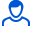Content: shpori.rar (1.06 MB)

Positive responses: 1
Negative responses: 0

Sold: 24
Refunds: 0Loyalty discount! If the total amount of your purchases from the seller more than:

 \$3 the discount is 10%

Spurs on higher mathematics (dif. Equations and series) check for yourself, all in a convenient form, certain formulas of the inserted picture. + To the entire set of tables with formulas in a gift !!! (Composition tables shown below ...)

Spurs consist of:

1. Diff. lv. 1st order (views)

2. The first order differential equations (general solution, particular solution, Theorem (existence and uniqueness of the solution of the problem of Cauchy-)

3. The problem of II cosmic speed

4. Wronskian (main theorem)

5. Lagrange equation

6. Symptom Leibniz

7. homogeneous linear equations with constant coefficients-of-u. (Euler's formula, Linear differential. Eq. Of higher order)

8. Theorem on a particular solution of the equation L [y] = f (x) on the right part in a special form

9. Signs of convergence znakopolozhit. series (the comparison test, the ultimate sign of comparison, d'Alembert's test, Cauchy)

10. T. (sufficient condition for the convergence of a number of belt-znakope

11. T. (Symptom Leibniz convergence, Abel)

12. Taylor series (Theorem)

13. Approximate value calculation function

14. T. (Dirichlet, Fourier series)

15. N-dimensional linear space

16. Euclidean space. (Pythagorean Theorem.)

17. Cauchy-Bunyakovskii

18. Cauchy integral method

19. Systems of linear differential. equations

20. The method of variation (Lagrange). Decision-inhomo- family of linear systems

21. Power series

22. Several Taylor

23. Table of integrals

Composition tables formulas:

1. Derivatives

2. The integrals

3. Rows

4. Operator method

Visit the website www.helpos.narod.ru, here you can download or order others. Finished work.
No feedback yet